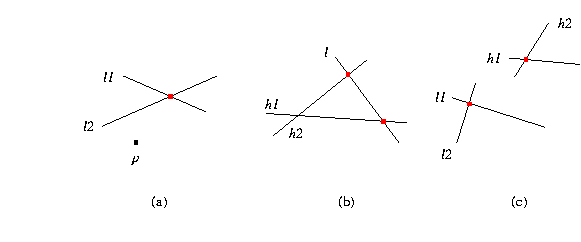## CGAL::compare_y

 Comparison_result compare_y ( Point_2 p, Point_2 q) compares Cartesian y-coordinates of p and q. Comparison_result compare_y ( Point_3 p, Point_3 q) compares Cartesian y-coordinates of p and q.Comparison_result compare_y ( Point_2 p, Line_2 l1, Line_2 l2) compares the y-coordinates of p and the intersection of lines l1 and l2, see (a) in the figure above. Comparison_result compare_y ( Line_2 l, Line_2 h1, Line_2 h2) compares the y-coordinates of the intersection of line l with line h1 and with line h2, see (b) in the figure above. Comparison_result compare_y ( Line_2 l1, Line_2 l2, Line_2 h1, Line_2 h2) compares the y-coordinates of the intersection of lines l1 and l2 and the intersection of lines h1 and h2 , see (c) in the figure above.

### See Also

CGAL::compare_xy
CGAL::compare_xyz
CGAL::compare_x
CGAL::compare_x_at_y
CGAL::compare_yx
CGAL::compare_y_at_x
CGAL::compare_z